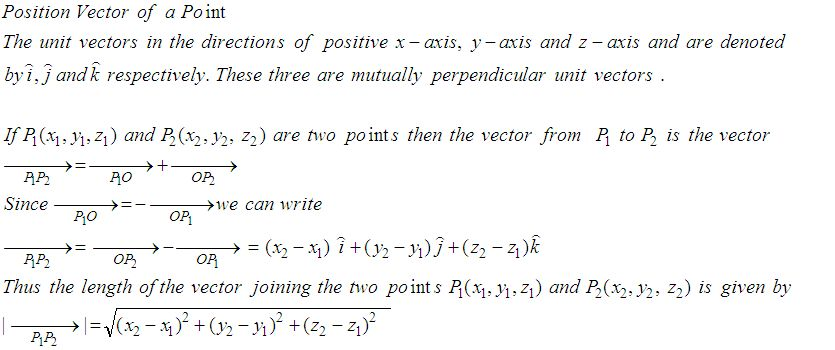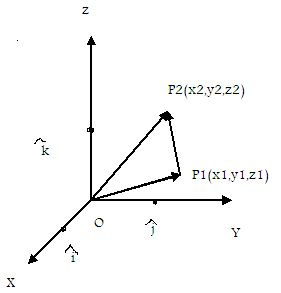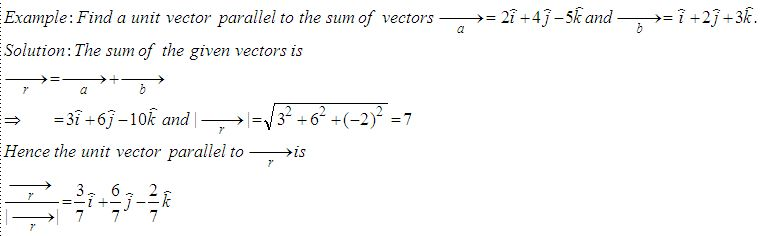Name: ___________________Date:___________________

 Email us to get an instant 20% discount on highly effective K-12 Math & English kwizNET Programs!

### High School Mathematics - 25.9 Position Vector of a PointDirections: Answer the following.Name: ___________________Date:___________________

### High School Mathematics - 25.9 Position Vector of a Point

 Q 1: If P = 2,4,7 and P2 = (-4, -1, 5) then find vector P1P2.-6i-5j-2k-6i+5j6i+5j+3k Q 2: Find the vector in the direction of the vector -i+2j+2k that has magnitude 7.-3i+2ji-j+k7(-i+2j+2k)/3 Q 3: Find a unit vector in the direction from P(3,2) towards Q(5,6).2i+34i+5i/Ö5 + 2j/Ö5 Q 4: Find the condition that the vectors a = ki + 3j and b = 4i+kj, (k not equal to 0) are parallel.k2 = 12k = 6k = 0 Q 5: Find the vector joining the initial point P(-4,2) and terminal point Q(0,-4) 8i-6j4i-6j4i+6j Q 6: Find a unit vector parallel to the sum of vectors a = 2i+4j-5k, b = i+2j+3k3/7i + 6/7j - 2/7 k8i+4j-3k2-i8 Q 7: Find the unit vector in the direction of of P(1,2) and Q(4,5).3i-2j(i+j)/Ö22i+5j Q 8: Find the unit vector in the direction of P(1,2,3) towards Q(4,5,6).(i+j+k)/Ö36i+5j+2k8j-2k Question 9: This question is available to subscribers only! Question 10: This question is available to subscribers only!